# Mass

Mass, in classical mechanics, is the measure of an object's resistance to change in motion, that is, its inertia, which is unchanging regardless of its changing gravitational context. On the surface of the earth the mass and weight of an object are essentially equivalent, but in a different gravitational context, such as in orbit around the earth, mass remains unchanged while weight becomes zero. In the framework of special relativity and general relativity, several different forms of mass are defined. One of these, the invariant mass, is close in concept to the classical idea of mass.

Probing into the depths of classical mechanics, physicists have conceptualized three subtly differentiated types of mass, which are important precisely because they have never been differentiated experimentally and that fact has become the basis of the equivalence principle, a pillar of the theory of general relativity. The three types of mass are:

• Inertial mass is a measure of an object's resistance to changing its state of motion when a force is applied. An object with small inertial mass changes its motion more readily, and an object with large inertial mass does so less readily.
• Passive gravitational mass is a measure of an object's response to being placed in a gravitational field. Within the same gravitational field, an object with a smaller passive gravitational mass experiences a smaller force than an object with a larger passive gravitational mass.
• Active gravitational mass is a measure of the strength of the gravitational field due to a particular object. For example, the gravitational field that one experiences on the Moon is weaker than that of the Earth because the Moon has less active gravitational mass.

Einstein developed his general theory of relativity by working on the assumption that the equivalence of inertial and gravitational masses is not accidental: That no experiment will ever detect a difference between them (the weak version of the equivalence principle) because "acceleration" (due to an external force) and "weight" (due to a gravitational field) are themselves identical.

## Introduction

Mass is the amount of matter and energy in a given object. One of the consequences of the equivalence of inertial mass and passive gravitational mass is the fact, famously demonstrated by Galileo Galilei, that objects with different masses fall at the same rate, assuming factors like air resistance are negligible. The theory of general relativity, the most accurate theory of gravitation known to physicists to date, rests on the assumption that inertial and passive gravitational mass are completely equivalent. This is known as the weak equivalence principle. Classically, active and passive gravitational mass were equivalent as a consequence of Newton's third law, but a new axiom is required in the context of relativity's reformulation of gravity and mechanics. Thus, standard general relativity also assumes the equivalence of inertial mass and active gravitational mass; this equivalence is sometimes called the strong equivalence principle.

If one were to treat inertial mass mi, passive gravitational mass mp, and active gravitational mass ma distinctly, Newton's law of universal gravitation would give as force on the second mass due to the first mass.$m_{i2}a_2=\frac{Gm_{a1}m_{p2}}{r^2}.$'

## Units of mass

In the SI system of units, mass is measured in kilograms (kg). Many other units of mass are also employed, such as: grams (g), tonnes, pounds, ounces, long and short tons, quintals, slugs, atomic mass units, Planck masses, solar masses, and eV/c2.

The eV/c2 unit is based on the electron volt (eV), which is normally used as a unit of energy. However, because of the relativistic connection between invariant mass and energy,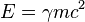$E =\gamma mc^2$ (see below), it is possible to use any unit of energy as a unit of mass instead. Thus, in particle physics where mass and energy are often interchanged, it is common to use not only eV/c2 but even simply eV as a unit of mass (roughly 1.783 × 10-36 kg). Masses are sometimes also expressed in terms of inverse lengths. Here one identifies the mass of a particle with its inverse Compton wavelength (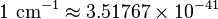$1 \mbox{ cm}^{-1}\approx 3.51767\times 10^{-41}$ kg).

Because the gravitational acceleration (g) is approximately constant on the surface of the Earth, and also because mass-balances do not depend on the local value of g, a unit like the pound is often used to measure either mass or force (for example, weight). When the pound is used as a measure of mass (where g does not enter in), it is officially in the English system defined in terms of the kg, as 1 lb = 0.453 592 37 kg (see force). In this case the English system unit of force is the poundal. By contrast, when the pound is used as the unit of force, the English unit of mass is the slug (mass).

For more information on the different units of mass, see Orders of magnitude (mass).

## Inertial mass

Inertial mass is the mass of an object measured by its resistance to acceleration.

To understand what the inertial mass of a body is, one begins with classical mechanics and Newton's Laws of Motion. Later on, we will see how our classical definition of mass must be altered if we take into consideration the theory of special relativity, which is more accurate than classical mechanics. However, the implications of special relativity will not change the meaning of "mass" in any essential way.

According to Newton's second law, we say that a body has a mass m if, at any instant of time, it obeys the equation of motion$f = \frac{\mathrm{d}}{\mathrm{d}t} (mv)$

where f is the force acting on the body and v is its velocity. For the moment, we will put aside the question of what "force acting on the body" actually means.

Now, suppose that the mass of the body in question is a constant. This assumption, known as the conservation of mass, rests on the ideas that (i) mass is a measure of the amount of matter contained in a body, and (ii) matter can never be created or destroyed, only split up or recombined. These are very reasonable assumptions for everyday objects, though, as we will see, the situation gets more complicated when we take special relativity into account. Another point to note is that, even in classical mechanics, it is sometimes useful to treat the mass of an object as changing with time. For example, the mass of a rocket decreases as the rocket fires. However, this is an approximation, based on ignoring pieces of matter which enter or leave the system. In the case of the rocket, these pieces correspond to the ejected propellant; if we were to measure the total mass of the rocket and its propellant, we would find that it is conserved.

When the mass of a body is constant, Newton's second law becomes$f = m \frac{\mathrm{d}v}{\mathrm{d}t} = m a$

where a denotes the acceleration of the body.

This equation illustrates how mass relates to the inertia of a body. Consider two objects with different masses. If we apply an identical force to each, the object with a bigger mass will experience a smaller acceleration, and the object with a smaller mass will experience a bigger acceleration. We might say that the larger mass exerts a greater "resistance" to changing its state of motion in response to the force.

However, this notion of applying "identical" forces to different objects brings us back to the fact that we have not really defined what a force is. We can sidestep this difficulty with the help of Newton's third law, which states that if one object exerts a force on a second object, it will experience an equal and opposite force. To be precise, suppose we have two objects A and B, with constant inertial masses mA and mB. We isolate the two objects from all other physical influences, so that the only forces present are the force exerted on A by B, which we denote fAB, and the force exerted on B by A, which we denote fBA. As we have seen, Newton's second law states that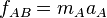$f_{AB} = m_A a_A \,$ and$f_{BA} = m_B a_B \,$

where aA and aB are the accelerations of A and B respectively. Suppose that these accelerations are non-zero, so that the forces between the two objects are non-zero. This occurs, for example, if the two objects are in the process of colliding with one another. Newton's third law then states that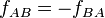$f_{AB} = - f_{BA} \,$

Substituting this into the previous equations, we obtain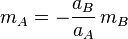$m_A = - \frac{a_B}{a_A} \, m_B$

Note that our requirement that aA be non-zero ensures that the fraction is well-defined.

This is, in principle, how we would measure the inertial mass of an object. We choose a "reference" object and define its mass mB as (say) 1 kilogram. Then we can measure the mass of every other object in the universe by colliding it with the reference object and measuring the accelerations.

## Gravitational mass

Gravitational mass is the mass of an object measured using the effect of a gravitational field on the object.

The concept of gravitational mass rests on Newton's law of gravitation. Let us suppose we have two objects A and B, separated by a distance |rAB|. The law of gravitation states that if A and B have gravitational masses MA and MB respectively, then each object exerts a gravitational force on the other, of magnitude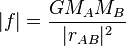$|f| = {G M_A M_B \over |r_{AB}|^2}$

where G is the universal gravitational constant. The above statement may be reformulated in the following way: If g is the acceleration of a reference mass at a given location in a gravitational field, then the gravitational force on an object with gravitational mass M is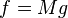$f = Mg \,$

This is the basis by which masses are determined by weighing. In simple bathroom scales, for example, the force f is proportional to the displacement of the spring beneath the weighing pan (see Hooke's law), and the scales are calibrated to take g into account, allowing the mass M to be read off. Note that a balance (see the subheading within Weighing scale) as used in the laboratory or the health club measures gravitational mass; only the spring scale measures weight.

## Equivalence of inertial and gravitational masses

The equivalence of inertial and gravitational masses is sometimes referred to as the Galilean equivalence principle or weak equivalence principle. The most important consequence of this equivalence principle applies to freely falling objects. Suppose we have an object with inertial and gravitational masses m and M respectively. If the only force acting on the object comes from a gravitational field g, combining Newton's second law and the gravitational law yields the acceleration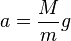$a = \frac{M}{m} g$

This says that the ratio of gravitational to inertial mass of any object is equal to some constant K if and only if all objects fall at the same rate in a given gravitational field. This phenomenon is referred to as the universality of free-fall. (In addition, the constant K can be taken to be 1 by defining our units appropriately.)

The first experiments demonstrating the universality of free-fall were conducted by Galileo. It is commonly stated that Galileo obtained his results by dropping objects from the Leaning Tower of Pisa, but this is most likely apocryphal; actually, he performed his experiments with balls rolling down inclined planes. Increasingly precise experiments have been performed, such as those performed by Loránd Eötvös, using the torsion balance pendulum, in 1889. To date, no deviation from universality, and thus from Galilean equivalence, has ever been found, at least to the accuracy 1/1012. More precise experimental efforts are still being carried out.

The universality of free-fall only applies to systems in which gravity is the only acting force. All other forces, especially friction and air resistance, must be absent or at least negligible. For example, if a hammer and a feather are dropped from the same height on Earth, the feather will take much longer to reach the ground; the feather is not really in free-fall because the force of air resistance upwards against the feather is comparable to the downward force of gravity. On the other hand, if the experiment is performed in a vacuum, in which there is no air resistance, the hammer and the feather should hit the ground at exactly the same time (assuming the acceleration of both objects towards each other, and of the ground towards both objects, for its own part, is negligible). This demonstration was, in fact, carried out in 1971 during the Apollo 15 Moonwalk, by Commander David Scott.

A stronger version of the equivalence principle, known as the Einstein equivalence principle or the strong equivalence principle, lies at the heart of the general theory of relativity. Einstein's equivalence principle states that it is impossible to distinguish between a uniform acceleration and a uniform gravitational field. Thus, the theory postulates that inertial and gravitational masses are fundamentally the same thing. All of the predictions of general relativity, such as the curvature of spacetime, are ultimately derived from this principle.

## Relativistic relation among mass, energy and momentum

Special relativity is a necessary extension of classical physics. In particular, special relativity succeeds where classical mechanics fails badly in describing objects moving at speeds close to the speed of light. One concept that needs to be clarified before we go any further is that of frames of reference. A frame of reference is where an observer happens to be, an example being you, the reader, sitting at your computer (your frame of reference). Even though you think that you are stationary, you are actually moving along with the earth in its rotation about its axis and revolution around the sun.

In relativistic mechanics, the invariant mass (m) of a free particle is related to its energy (E) and momentum (p) by the equation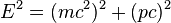$E^2 = (mc ^ 2) ^ 2 + (pc) ^ 2 \,$

where c is the speed of light. This is sometimes referred to as the mass-energy-momentum equation.

The invariant mass is also referred to as rest mass and is the mass of an particle at rest, with respect to the observer. Rest mass is independent of the reference frame as it is an particle's inherent mass (i.e. it is constant).

In the rest frame, the velocity is zero, and thus so is the momentum p. The mass-energy-momentum relation thus reduces to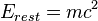$E_{rest} = mc^2 \,$

This is the rest energy of an particle and, like rest mass, it is a constant for that particle (for example, the rest energy of an electron is 0.511MeV). This equation is important because it tells us that rest mass is simply one form of energy. Even a small mass can release an enormous amount of energy because the c in the equation is a very big number (669,600,000 miles/hour) The significance of this is that any change in the rest mass is followed by a change in the energy. In nuclear fusion, two atoms are placed in an extremely high temperature environment, they fuse together, yet the mass of the resulting fused atom is less than the mass of the two component atoms. The missing mass is released as some form of outward energy, such as heat. This is the principle behind atomic bombs, where the missing mass is converted into destructive energy. For massless particles(m=0), the mass-energy-momentum equation simplifies to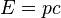$E = pc \,$

In classical mechanics, massless objects are an ill-defined concept, since applying any force to one would produce, via Newton's second law, an infinite acceleration. In relativistic mechanics, they are objects that are always traveling at the speed of light, an example being light itself, in the form of photons. The above equation says that the energy carried by a massless object is directly proportional to its momentum.